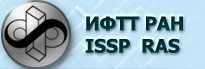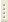06.02.2023Measurement Basis

Prof. V.T.Dolgopolov

Curriculum

1. Introduction. Types of measurement. Definitions and standards. Units and systems of units.
2. Methods of measurement. Measurement strategy. Measurement errors.
3. Characteristics of measurement systems. Description of measurement systems using differential equations.
4. Reciprocal effect of measurement system on measured object, matching. Noise and disturbance (interference).
5. Structure of measurement systems. Sensors.
6. Measured signal transform. Ideal operational amplifier.
7. Specific circuits on operational amplifiers. Lock-in amplifier. Nonlinear signal processing.
8. Digital-to-analog conversion. Digital-to-analog converters.
9. Instrument-computer data exchange.  Slang. Tasks.

Note.  The course does not include estimation of systematic and random errors as it is assumed to be known from laboratory practice at Institute of Physics and Technology.